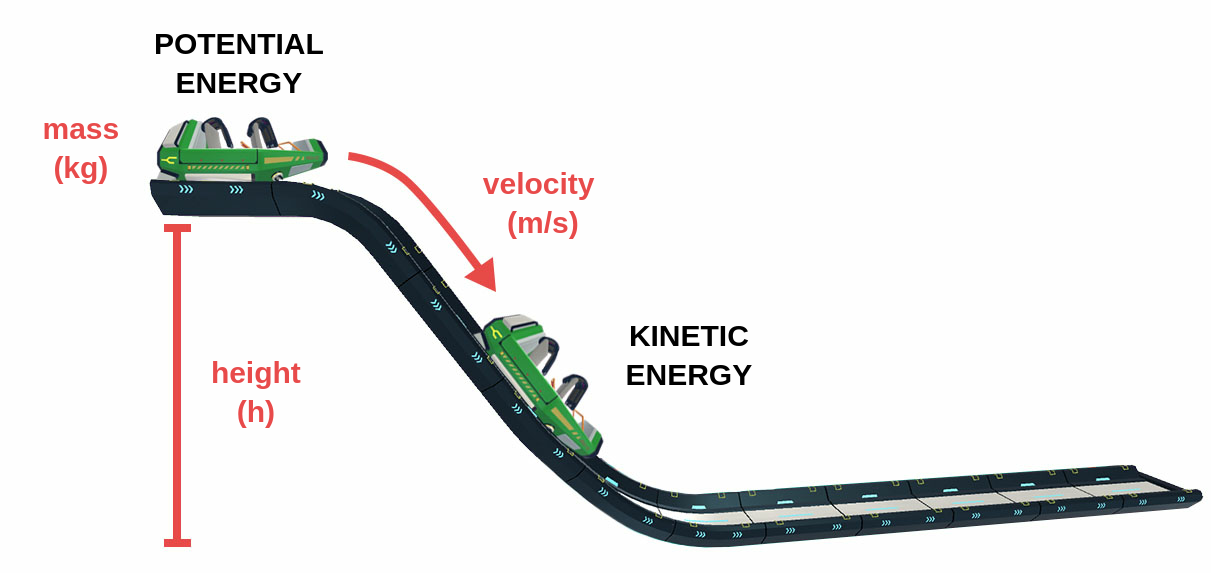Kinetic energy is the energy an object possesses due to its motion. It is defined as the work necessary to accelerate a body of a given mass from rest to a given velocity. Kinetic energy, as shown by the formula below, is one half the product of mass (m) and velocity squared (v2). Kinetic energy is measured in joules (kg·m2/s2).

### EK = 1/2 m v2

For example, when a rock containing potential energy due to being high on a cliff falls, its potential energy is converted into kinetic energy while the rock falls to the ground. Similarly, when a car is on a highway, the chemical energy in the car's fuel is converted into the kinetic energy of the car's motion. Any moving body maintains a constant kinetic energy as long as its speed does not change. Together, potential energy and kinetic energy make up mechanical energy.Figure 1: Potential and Kinetic Energy